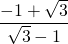# 9.5 Rationalizing Denominators

It is considered non-conventional to have a radical in the denominator. When this happens, generally the numerator and denominator are multiplied by the same factors to remove the radical denominator. The problems in the previous section dealt with removing a monomial radical. In this section, the previous strategy is expanded to include binomial radicals.

Example 9.5.1

Rationalize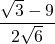.

To rationalize the denominator,  multiply out the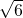.

This will look like: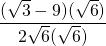Multiplying thethroughout yields: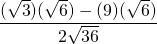Which reduces to: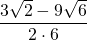And simplifies to: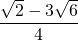Please note that, in reducing the numerator and denominator by the factor 3, reduce each term in the numerator by 3.

Quite often, there will be a denominator binomial that contains radicals. For these problems, it is easiest to use a feature from the sum and difference of squares: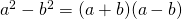.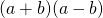are termed conjugates of each other. They are identical binomials, except that their signs are opposite. When encountering radical binomials, simply multiply by the conjugates to square out the radical.

Example 9.5.2

Square out the radical of the binomial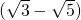using its conjugate.

The conjugate ofis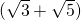.

When multiplied, these conjugates yield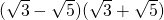or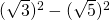.

This yields 3 − 5 = −2.

When encountering a radicalized binomial denominator, the best solution is to multiply both the numerator and denominator by the conjugate of the denominator.

Example 9.5.3

Rationalize the denominator of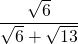.

Multiplying the numerator and denominator by the denominator’s conjugate yields: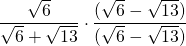When multiplied out, this yields: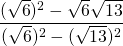Which reduces to: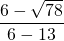Or: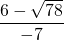# Questions

Rationalize the following radical fractions.

1.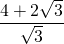2.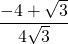3.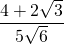4.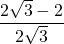5.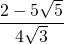6.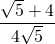7.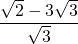8.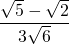9.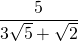10.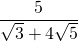11.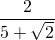12.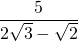13.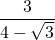14.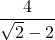15.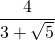16.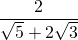17.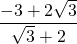18.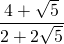19.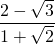20.